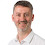## Monday 10 August 2009

### Sets =- or -=???

This question arose recently: Note: Using gt and lt for Greater Than and Less Than symbols - sorry. What is the difference between the set { < Value = -{"X"} > } and the set { < Value -= {"X"} > }? Well the difference is that the first set is a modification of the \$ set and saying that I want all values of the "Value" field excluding the value "X" (it could have also been written as { < Value = {*}-{"X"} > ). The second set is different in that it is additive (or actually subtractive) - it will take whatever selections are on the "Value" field and take off the "X". So, for the first one, if you make any selections on the "Value" field, they will be ignored. For the second one, your selections will be reflected in the result except if "X" is part of your selections it will be excluded. As an example, take the following dataset: Sales: Load * Inline [ User, Sales John, 12222 Tom, 11000 Jane, 19000 May, 21000 Graham, 13300 ]; If I create a straight table with User as the dimension and the following 2 expressions: Sum({ < User = -{"John"} > Sales) Sum({ < User -= {"John"} > Sales) Then whatever selection I make on User will not effect the result - the chart will list the sales for Time, Jane, May and Graham (no John). For the second, if I make selections on a user, the other users will zero out but I will still see no John. Enjoy.

1.Stephen,

We have actually been trying to do the same thing. I used your example above, but no success. This is what we were trying to do. I guess we were close, but no cigar.

Any ideas why above syntax is not working. I thought it was due a missing } at the end, but still no results.
Here is the scenario. In a straight table, trying to sum all the inventory based on the current selection but with one criteria element, the Distributor, being the opposite of what is currently selected. For example, Fiscal Year is 2009. Month is 4 and Distributor is Arrow. Want to see totals for the first two and everything except arrow. For what its worth, distributor is one of the tables dimensions.

sum( {\$ltDistributor= ~{\$(Distributor)}gt} (End_Qty + EndX_Qty) )

sum( {\$ltDistributor -= {\$(Distributor)}gt} (End_Qty + EndX_Qty) )

2.Hi,

Here is where you need to use a function like Concat to correctly return the list of selected values from Distributor:

=sum( {\$<Distributor = -{\$(=Concat(Distributor,','))}>} (End_Qty + EndX_Qty) )

3.hi stephen,

lt or gt is not recognized by qlikview? are they pre-defined sets? or do we have to define them seperately?

4.gt = greater than = >
lt = less than = <

when I wrote this post, it did not accept the http "& lt ;"

5.Hi Stephen,

I would like to use this same logic, but do a count on the # of distribotors that make the End_Qty + EndX_Qty. I do not want to include the selected distributor in my calculation. Any suggestions?